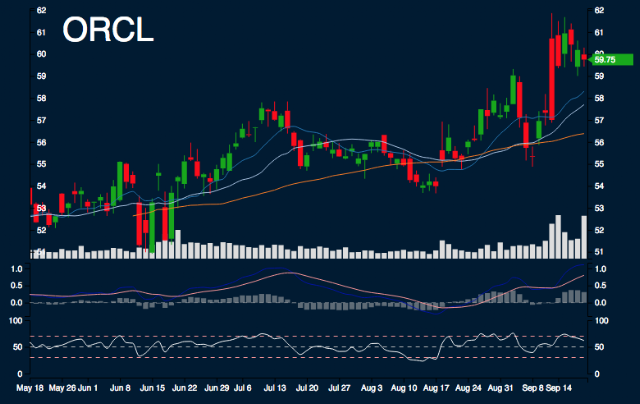#ORCL Report

Sample Size: 10; Percent Error: 15%

## Current Trend Performance

ORCL is -1.99% from monthly high, 9.64% from monthly low, 2.47% from 10 day simple moving average, 3.56% from 20 day simple moving average, and 5.98% from 50 day simple moving average.

## Forecast Ranges

Day 1:
The highs are 61.33, the lows are 58.38, and the median is 59.86. 37.5% to decrease between -3.32% - 0%. 62.5% to increase between 0% - 3.32%.
Day 2:
The highs are 61.01, the lows are 57.96, and the median is 59.48. 44.44% to decrease between -3.32% - 0%. 55.56% to increase between 0% - 3.32%.
Day 3:
The highs are 61.41, the lows are 56.25, and the median is 58.83. 11.11% to decrease between -6.64% - -3.32%. 33.33% to decrease between -3.32% - 0%. 55.56% to increase between 0% - 3.32%.
Day 4:
The highs are 60.68, the lows are 55.59, and the median is 58.14. 11.11% to decrease between -9.96% - -6.64%. 44.44% to decrease between -3.32% - 0%. 44.44% to increase between 0% - 3.32%.
Day 5:
The highs are 60.55, the lows are 56.14, and the median is 58.34. 22.22% to decrease between -6.64% - -3.32%. 44.44% to decrease between -3.32% - 0%. 33.33% to increase between 0% - 3.32%.
Day 6:
The highs are 60.76, the lows are 56.25, and the median is 58.5. 33.33% to decrease between -6.64% - -3.32%. 22.22% to decrease between -3.32% - 0%. 44.44% to increase between 0% - 3.32%.
Day 7:
The highs are 60.67, the lows are 55.46, and the median is 58.06. 11.11% to decrease between -9.96% - -6.64%. 22.22% to decrease between -6.64% - -3.32%. 44.44% to decrease between -3.32% - 0%. 22.22% to increase between 0% - 3.32%.

## Summary

SMA: Simple Moving Average

In next 5 days, there is possible support range between 57.77 and 58.43, because of 20 day SMA. There is possible resistance range between 60.41, because of monthly high, and 61.73. Areas of uncertainty are beyond 57.13 and beyond 63.1. Minimum movement range is 3.32%.

Research conducted with Nforecast.

This is not a recommendation to buy, sell, or hold. This research is for educational, informational, and entertainment purposes. You should not construe any data or information presented by members on this blog or Nforecast LLC as investment, tax, legal, financial or other advice.